# ILP Part 15 – Basic set operations

This is the fifteenth part of the ILP series. For your convenience you can find other parts in the table of contents in Part 1 – Boolean algebra

Today we are going to use comparisons to implement few basic set operations. Let’s go.

# In set, Not in set

We start with In set operation. For set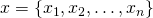and value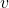we want to ask whetheris in set. This is very easy because all we need to do is comparewith all values from set and calculate the sum: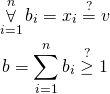For every value we calculate variable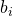which is true when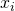is equal to. Next, we sum all values and check whether the sum is positive. If it is true then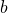is true which means thatis in set.
For operation Not in set we do almost the same. The only difference is in comparing the sum with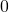.

# Different values count

Very similar operation is a Different values count. For multiset (e.g., set with repetitions)we want to ask how many different values does it contain. The formula is as follows: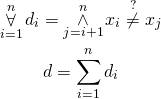For every variablewe check whether all variables with indexes greater thanare different than. Basically, we traverse multisetin some arbitrary order and for-th value we check whether all remaining values are different.

# All different

We can use previous formula to make sure that all values in mulitset are different (so the multiset is in fact set). To achieve that we need to calculate different values count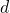and add constraint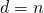whereis a number of variables in a multiset.

# Summary

Today we implemented useful set operations. Next time we are going to utilize all ILP knowledge to solve real problem — we will be solving a simple cube riddle.